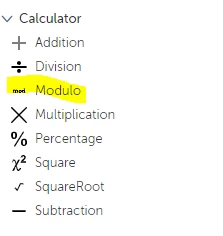Question

# How we can use modulus (i.e %) operator in A360? Actually i want to check whether a entered number is whole number or decimal number and the logic is num % 1 != 0. e.g 15%1=0 15.5%1=0.5

• 228 views• Partner
• 0 replies
How we can use modulus (i.eoperator in A360? Actually i want to check whether a entered number is whole number or decimal number and the logic is num % 1 != 0. e.g 15%1=0 15.5%1=0.5😊